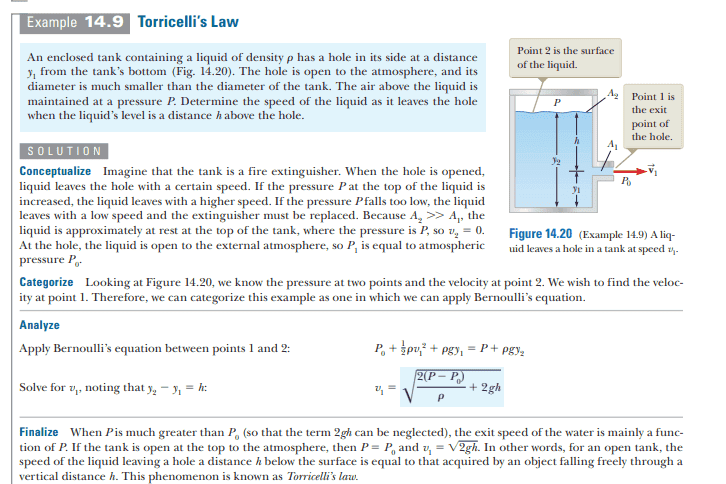# When is Torricelli’s Law an Approximation?

Callumnc1
Homework Statement:
An enclosed tank containing a liquid of density row has a hole in its side at a distance y1
from the tank’s bottom (Fig. 14.20). The hole is open to the atmosphere, and its
diameter is much smaller than the diameter of the tank. The air above the liquid is
maintained at a pressure P. Determine the speed of the liquid as it leaves the hole
when the liquid’s level is a distance h above the hole.
Relevant Equations:
Bernoulli’s equation
Hi!

For this problem,When Area 2 > Area 1, but not by much, is this phenomenon no longer called Torricelli's Law because the water is not approximately stationary at the top surface? What is this called now?

Many thanks!

Last edited by a moderator:

Homework Helper
Gold Member
2022 Award
When Area 2 > Area 1, but not by much, is this phenomenon no longer called Torricelli's Law because the water is not approximately stationary at the top surface? What is this called now?
Torricelli's Law is the approximation of Bernoulli's equation when the source area is much greater than the exit area. So if you are not making that approximation it is called ... what do you think?

•Callumnc1
Callumnc1
Torricelli's Law is the approximation of Bernoulli's equation when the source area is much greater than the exit area. So if you are not making that approximation it is called ... what do you think?
Bernoulli's equation? I guess there is no name for the general case.

Many thanks!

Homework Helper
Gold Member
2022 Award
•Callumnc1 and Lnewqban
Callumnc1
•Lnewqban